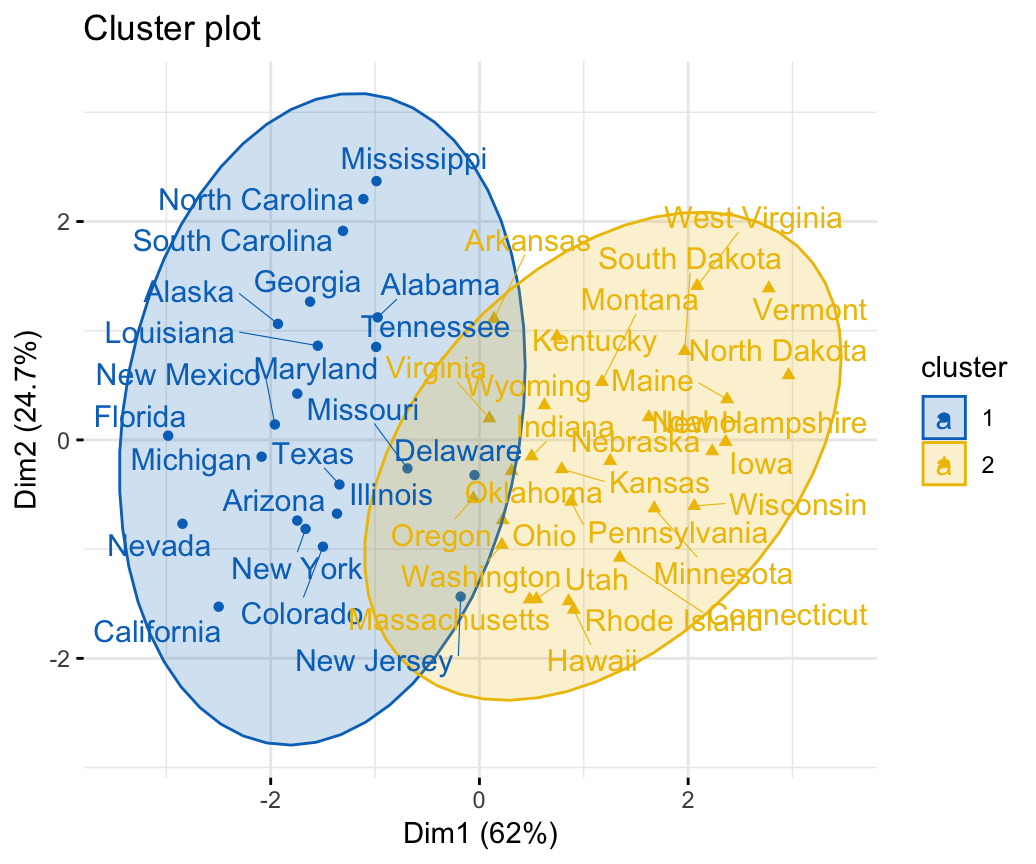## Fuzzy Clustering Essentials

The fuzzy clustering is considered as soft clustering, in which each element has a probability of belonging to each cluster. In other words, each element has a set of membership coefficients corresponding to the degree of being in a given cluster.

This is different from k-means and k-medoid clustering, where each object is affected exactly to one cluster. K-means and k-medoids clustering are known as hard or non-fuzzy clustering.

In fuzzy clustering, points close to the center of a cluster, may be in the cluster to a higher degree than points in the edge of a cluster. The degree, to which an element belongs to a given cluster, is a numerical value varying from 0 to 1.

The fuzzy c-means (FCM) algorithm is one of the most widely used fuzzy clustering algorithms. The centroid of a cluster is calculated as the mean of all points, weighted by their degree of belonging to the cluster:

In this article, we’ll describe how to compute fuzzy clustering using the R software.

#### Related Book

Practical Guide to Cluster Analysis in R

## Required R packages

We’ll use the following R packages: 1) cluster for computing fuzzy clustering and 2) factoextra for visualizing clusters.

## Computing fuzzy clustering

The function fanny() [cluster R package] can be used to compute fuzzy clustering. FANNY stands for fuzzy analysis clustering. A simplified format is:

fanny(x, k, metric = "euclidean", stand = FALSE)
• x: A data matrix or data frame or dissimilarity matrix
• k: The desired number of clusters to be generated
• metric: Metric for calculating dissimilarities between observations
• stand: If TRUE, variables are standardized before calculating the dissimilarities

The function fanny() returns an object including the following components:

• membership: matrix containing the degree to which each observation belongs to a given cluster. Column names are the clusters and rows are observations
• coeff: Dunn’s partition coefficient F(k) of the clustering, where k is the number of clusters. F(k) is the sum of all squared membership coefficients, divided by the number of observations. Its value is between 1/k and 1. The normalized form of the coefficient is also given. It is defined as $(F(k) - 1/k) / (1 - 1/k)$, and ranges between 0 and 1. A low value of Dunn’s coefficient indicates a very fuzzy clustering, whereas a value close to 1 indicates a near-crisp clustering.
• clustering: the clustering vector containing the nearest crisp grouping of observations

For example, the R code below applies fuzzy clustering on the USArrests data set:

library(cluster)
df <- scale(USArrests)     # Standardize the data
res.fanny <- fanny(df, 2)  # Compute fuzzy clustering with k = 2

The different components can be extracted using the code below:

head(res.fanny$membership, 3) # Membership coefficients ## [,1] [,2] ## Alabama 0.664 0.336 ## Alaska 0.610 0.390 ## Arizona 0.686 0.314 res.fanny$coeff # Dunn's partition coefficient
## dunn_coeff normalized
##      0.555      0.109
head(res.fanny\$clustering) # Observation groups
##    Alabama     Alaska    Arizona   Arkansas California   Colorado
##          1          1          1          2          1          1

To visualize observation groups, use the function fviz_cluster() [factoextra package]:

library(factoextra)
fviz_cluster(res.fanny, ellipse.type = "norm", repel = TRUE,
palette = "jco", ggtheme = theme_minimal(),
legend = "right")To evaluate the goodnesss of the clustering results, plot the silhouette coefficient as follow:

fviz_silhouette(res.fanny, palette = "jco",
ggtheme = theme_minimal())
##   cluster size ave.sil.width
## 1       1   22          0.32
## 2       2   28          0.44## Summary

Fuzzy clustering is an alternative to k-means clustering, where each data point has membership coefficient to each cluster. Here, we demonstrated how to compute and visualize fuzzy clustering using the combination of cluster and factoextra R packages.